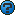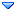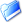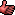GSI Forum
GSI Helmholtzzentrum für Schwerionenforschung

Home » PANDA » PandaRoot » Bugs, Fixes, Releases » negative masses in geometry (TG3)negative masses in geometry (TG3) Wed, 20 April 2011 14:36Olaf Hartmann Messages: 105Registered: December 2003 Location: Wien-Alsergrund continuous participant From: *smi.oeaw.ac.at
Dear all,

when calculating the masses of the volumes in PandaRoot, I observed i.a. several masses with negative values, which actually comes from a negative volume size (!). Example:

Calculating the physical volume (which for a TGeoXtru is [length]³):
```root  gGeoManager->GetVolume("Supporto2")->Capacity()
(const Double_t)(-4.86071999981845693e+03)
```

These are the geo parameters of this object:
```root  gGeoManager->GetVolume("Supporto2")->InspectShape()
*** Shape Supporto2: TGeoXtru ***
Nz    = 2
List of (x,y) of polygon vertices:
x =   134.00000  y =   184.00000
x =   130.51000  y =   184.00000
x =   130.51000  y =   176.00000
x =   134.00000  y =   176.00000
x =   134.00000  y =   178.00000
x =   156.70000  y =   178.00000
x =   156.70000  y =   176.00000
x =   159.20000  y =   176.00000
x =   159.20000  y =   184.00000
x =   156.70000  y =   184.00000
x =   156.70000  y =   182.00000
x =   134.00000  y =   182.00000
plane 0: z=   -0.00000 x0=    0.00000 y0=    0.00000 scale=    1.00000
plane 1: z=    2.00000 x0=    0.00000 y0=    0.00000 scale=    1.00000
Bounding box:
*** Shape Supporto2: TGeoBBox ***
dX =    14.34500
dY =     4.00000
dZ =     1.00000
origin: x=  144.85500 y=  180.00000 z=    1.00000
```

Shouldn't it be that values like volume and mass always come out with positive values?
Of course I can ask for the abs value in the code to avoid negative values.

Cheers
Olaf.negative masses in geometry (TG3) By: Olaf Hartmann on Wed, 20 April 2011 14:36Re: negative masses in geometry (TG3) By: Mohammad Al-Turany on Wed, 20 April 2011 15:19Re: negative masses in geometry (TG3) By: Olaf Hartmann on Wed, 20 April 2011 16:10Re: negative masses in geometry (TG3) By: Mohammad Al-Turany on Wed, 20 April 2011 22:56Re: negative masses in geometry (TG3) By: StefanoSpataro on Thu, 21 April 2011 08:00Re: negative masses in geometry (TG3) By: Olaf Hartmann on Thu, 21 April 2011 08:35Re: negative masses in geometry (TG3) By: Olaf Hartmann on Thu, 21 April 2011 10:26
 Previous Topic: TPC compilation error Next Topic: STT tracking crash during check getMCInfo.
Goto Forum:

Current Time: Thu Feb 02 10:19:34 CET 2023

Total time taken to generate the page: 0.01064 seconds